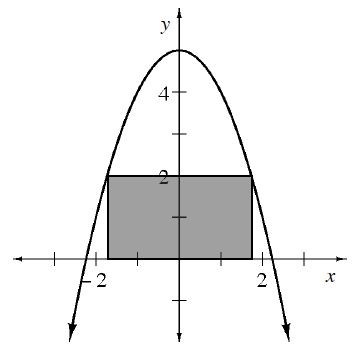### Home > CALC3RD > Chapter Ch5 > Lesson 5.1.4 > Problem5-40

5-40.

A rectangle is bounded by the function $y = –x^{2} + 5$ and the $x$-axis as shown below.1. If the base of the rectangle is $2x$, what is the height?

Notice that the rectangle is symmetric across the $y$-axis.

Because of the symmetry, the endpoints of the rectangle can be found at $−x$ and $+x...$ after all, the distance between $−x$ and $x$ is $2x$, the length of the base.

Consequently, the height of the rectangle can be evaluated at $f\left(x\right)$:
height $= y = −x^{2} + 5$

2. What is the maximum area that the rectangle can enclose?

Area = (base)(height)
Use the base and height you found in part (a).Then optimize the Area function.

To find the maximum Area:
1. Let $A^\prime\left(x\right) = 0$.
2. Solve for $x$.
3. Use the $x$ and your expressions for base & height to calculate the maximum area.

3. If you make the rectangle from part (b) into a flag and spin it around the $x$-axis, what is the resulting volume?

A cylinder with height __________ and radius __________.

4. Determine the value of $x$ so that the volume of the rotated rectangle is a maximum.

Volume of a cylinder: $V = πr^{2}h$
If you need guidance optimizing the volume, refer to steps in part (b).